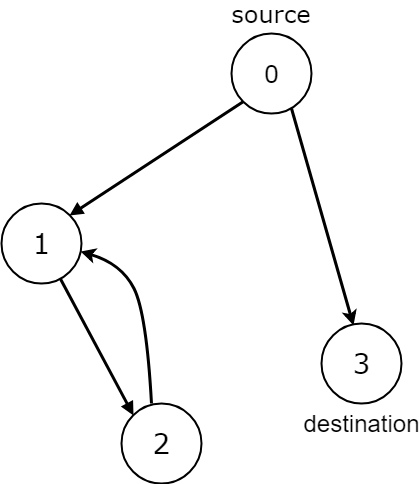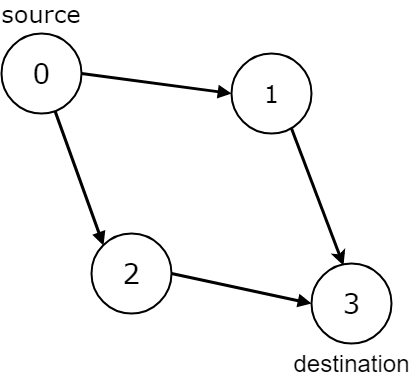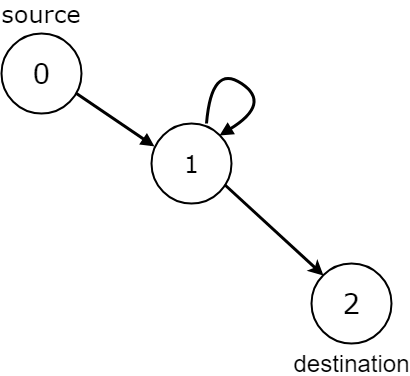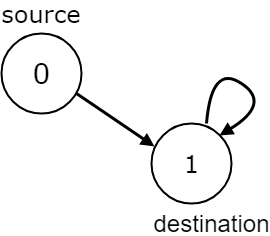1059. All Paths from Source Lead to Destination

Given the `edges` of a directed graph where `edges[i] = [ai, bi]` indicates there is an edge between nodes `ai` and `bi`, and two nodes `source` and `destination` of this graph, determine whether or not all paths starting from `source` eventually, end at `destination`, that is:

• At least one path exists from the `source` node to the `destination` node
• If a path exists from the `source` node to a node with no outgoing edges, then that node is equal to `destination`.
• The number of possible paths from `source` to `destination` is a finite number.

Return `true` if and only if all roads from `source` lead to `destination`.

Example 1:```Input: n = 3, edges = [[0,1],[0,2]], source = 0, destination = 2
Output: false
Explanation: It is possible to reach and get stuck on both node 1 and node 2.
```

Example 2:```Input: n = 4, edges = [[0,1],[0,3],[1,2],[2,1]], source = 0, destination = 3
Output: false
Explanation: We have two possibilities: to end at node 3, or to loop over node 1 and node 2 indefinitely.
```

Example 3:```Input: n = 4, edges = [[0,1],[0,2],[1,3],[2,3]], source = 0, destination = 3
Output: true
```

Example 4:```Input: n = 3, edges = [[0,1],[1,1],[1,2]], source = 0, destination = 2
Output: false
Explanation: All paths from the source node end at the destination node, but there are an infinite number of paths, such as 0-1-2, 0-1-1-2, 0-1-1-1-2, 0-1-1-1-1-2, and so on.
```

Example 5:```Input: n = 2, edges = [[0,1],[1,1]], source = 0, destination = 1
Output: false
Explanation: There is infinite self-loop at destination node.
```

Constraints:

• `1 <= n <= 104`
• `0 <= edges.length <= 104`
• `edges.length == 2`
• `0 <= ai, bi <= n - 1`
• `0 <= source <= n - 1`
• `0 <= destination <= n - 1`
• The given graph may have self-loops and parallel edges.

1059. All Paths from Source Lead to Destination
``````struct Solution;
impl Solution {
fn leads_to_destination(n: i32, edges: Vec<Vec<i32>>, source: i32, destination: i32) -> bool {
let n = n as usize;
let s = source as usize;
let d = destination as usize;
let mut visited: Vec<bool> = vec![false; n];
let mut graph: Vec<Vec<usize>> = vec![vec![]; n];
for edge in edges {
let u = edge as usize;
let v = edge as usize;
graph[u].push(v);
}
if !graph[d].is_empty() {
return false;
}
Self::dfs(s, &mut visited, &graph, d)
}

fn dfs(u: usize, visited: &mut [bool], graph: &[Vec<usize>], d: usize) -> bool {
if u == d {
true
} else {
if visited[u] {
false
} else {
visited[u] = true;
let mut count = 0;
for &v in &graph[u] {
if !Self::dfs(v, visited, graph, d) {
return false;
} else {
count += 1;
}
}
visited[u] = false;
count != 0
}
}
}
}

#[test]
fn test() {
let n = 3;
let edges = vec_vec_i32![[0, 1], [0, 2]];
let source = 0;
let destination = 2;
let res = false;
assert_eq!(
res
);
let n = 4;
let edges = vec_vec_i32![[0, 1], [0, 3], [1, 2], [2, 1]];
let source = 0;
let destination = 3;
let res = false;
assert_eq!(
res
);
let n = 4;
let edges = vec_vec_i32![[0, 1], [0, 2], [1, 3], [2, 3]];
let source = 0;
let destination = 3;
let res = true;
assert_eq!(
res
);
let n = 3;
let edges = vec_vec_i32![[0, 1], [1, 1], [1, 2]];
let source = 0;
let destination = 2;
let res = false;
assert_eq!(
res
);
let n = 2;
let edges = vec_vec_i32![[0, 1], [1, 1]];
let source = 0;
let destination = 1;
let res = false;
assert_eq!(